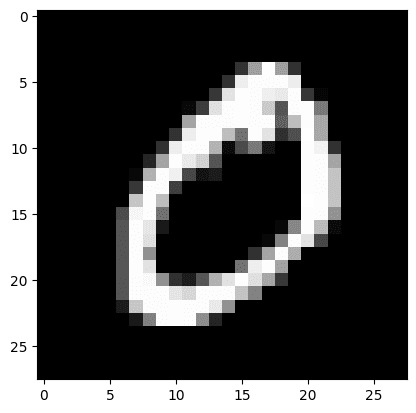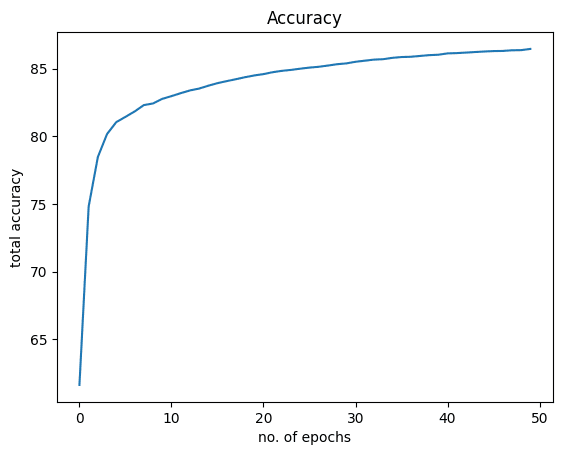# Building a Logistic Regression Classifier in PyTorch

Logistic regression is a type of regression that predicts the probability of an event. It is used for classification problems and has many applications in the fields of machine learning, artificial intelligence, and data mining.

The formula of logistic regression is to apply a sigmoid function to the output of a linear function. This article discusses how you can build a logistic regression classifier. While previously you have been working on a single-varable dataset, here we’ll be using a popular MNIST dataset to train and test our model. After going through this article, you’ll learn:

• How to use logistic regression in PyTorch and how it can be applied to real-world problems.
• How to load and analyze torchvision datasets.
• How to build and train a logistic regression classifier on image datasets.

Let’s get started.

Building a Logistic Regression Classifier in PyTorch.
Picture by Catgirlmutant. Some rights reserved.

## Overview

This tutorial is in four parts; they are

• The MNIST Dataset
• Build the Model with `nn.Module`
• Training the Classifier

## The MNIST Dataset

You will train and test a logistic regression model with MNIST dataset. This dataset contains 6000 images for training and 10000 images for testing the out-of-sample performance.

The MNIST dataset is so popular that it is part of PyTorch. Here is how you can load the training and testing samples of the MNIST dataset in PyTorch.

The dataset will be downloaded and extracted to the directory as below.

Let’s verify number of training and testing samples in the dataset.

It prints

Each sample in the dataset is a pair of image and label. To inspect the data type and size of the first element in the training data, you can use `type()` and `size()` methods.

This prints

You can access samples from a dataset using list indexing. The first sample in the dataset is a `FloatTensor` and it is a \$28times 28\$-pixel image in grayscale (i.e., one channel), hence the size `[1, 28, 28]`.

Now, let’s check the labels of the first two samples in the training set.

This shows

From the above, you can see that the first two images in the training set represent “5” and “0”. Let’s show these two images to confirm.

You should see these two digits:Usually, you do not use the dataset directly in training but through a `DataLoader` class. This allows you to read data in batches, not samples.

In the following, data is loaded into a `DataLoader` with batch size at 32.

Kick-start your project with my book Deep Learning with PyTorch. It provides self-study tutorials with working code.

## Build the Model with `nn.Module`

Let’s build the model class with `nn.Module` for our logistic regression model. This class is similar to that in the previous posts but the numbers of input and output are configurable.

This model will take a \$28times 28\$-pixel image of handwritten digits as input and classify them into one of the 10 output classes of digits 0 to 9. So, here is how you can instantiate the model.

## Training the Classifier

You will train this model with stochastic gradient descent as the optimizer with learning rate 0.001 and cross-entropy as the loss metric.

Then, the model is trained for 50 epochs. Note that you have use `view()` method to flatten the image matrices into rows to fit the same of the logistic regression model input.

During training, you should see the progress like the following:

You have achieved an accuracy of around 86% by training the model for only 50 epochs. Accuracy can be improved further if the model is trained longer.

Let’s visualize how the graphs for loss and accuracy look like. The following is the loss:And this is for accuracy:Putting everything together, the following is the complete code:

## Summary

In this tutorial, you learned how to build a multi-class logistic regression classifier in PyTorch. Particularly, you learned.

• How to use logistic regression in PyTorch and how it can be applied to real-world problems.
• How to load and analyze torchvision datasets.
• How to build and train a logistic regression classifier on image datasets.

## Get Started on Deep Learning with PyTorch!#### Learn how to build deep learning models

…using the newly released PyTorch 2.0 library

Discover how in my new Ebook:
Deep Learning with PyTorch

It provides self-study tutorials with hundreds of working code to turn you from a novice to expert. It equips you with
tensor operation, training, evaluation, hyperparameter optimization,
and much more…

#### Kick-start your deep learning journey with hands-on exercises

See What’s Inside